Volume 65 Supplement 11

# Determining field-aligned currents with the Swarm constellation mission

## Abstract

Field-aligned currents (FAC) are the prime mechanism for coupling energy from the solar wind into the upper atmosphere at high latitudes. Knowing their intensity and distribution is of pivotal importance for the selection of quiet time data at high latitudes to be used in main field analysis. At the same time FACs can be regarded as a key element for studies of magnetosphere-ionosphere interactions. The Swarm satellite constellation, in particular the lower pair, provides the opportunity to determine radial currents uniquely. The computation of FACs from the vector magnetic field data is a straightforward and fast process, applying Ampère’s integral law to a set of four magnetic field values. In this method the horizontal magnetic field components at a quad of measurement points sampled by the two satellites moving side-by-side are interpreted. The presented algorithm was implemented as described here in the Swarm Level-2 processing facility to provide the automatically estimated radial and field-aligned currents. It was tested with synthetic data in the Swarm Level-1b format. The resulting currents agree excellently with the input currents of the synthetic model. The data products are computed along the entire orbits. In addition, the L2 processor calculates also FACs with a 1 Hz time resolution individually from the three single Swarm satellites.

## 1. Introduction

Field aligned currents play an important role in space plasmas. They are able to transfer energy almost loss-less over large distances. In the magnetosphere, they connect distant source regions with the high-latitude ionosphere. There, they drive the entire auroral current system (Untiedt and Baumjohann, 1993). At lower latitudes, FACs flow whenever potential differences between the ionospheres of the two hemispheres build up. Rather well-known are the interhemispheric FACs connecting the foci of the two Sq (solar quiet) current systems (e.g. Fukushima, 1979; Park et al., 2011). To improve knowledge about these processes it would be desirable to have reliable measurements of the FACs in near-Earth space. One way to acquire this information is by performing closely-spaced multi-point magnetic field measurements. ESA’s Swarm constellation mission provides this opportunity.

A constellation of three satellites can do more than three single satellites. In this sense, the purpose of the Swarm Level-2 Processor is to provide advanced data products that take advantage of the dedicated constellation of three Swarm satellites (Olsen et al., 2013). Field-aligned currents computed along track from the magnetic field measured at single satellites have always suffered from non-uniqueness. Since the satellite moves through three-dimensional regions of high current density, the recorded field changes can be interpreted in terms of current density only if certain assumptions on the current geometry and its stationarity are made (Lühr et al., 1996). With measurements being available only along the orbit direction, i.e. along-track, the current distribution has to be generally assumed constant over the time span of passage and organized in sheets of known orientation (Lühr et al., 1996; Stauning et al., 2001).

More realistic FAC densities can be computed directly and uniquely from magnetic field measurements if synchronous, multi-point measurements spanning a two-dimensional area in space are available. The two lower Swarm satellites flying side-by-side provide this type of datasets. The benefit of constellation processing for the determination of field-aligned currents has been demonstrated in an ESA-sponsored scientific study during Swarm Mission Phase A (Vennerstrøm et al., 2005; Ritter and Lühr, 2006). With the planned constellation of three satellites in near-polar orbits (inclination ~87°) at two different heights, one at 530 km and a pair at initially 460 km (Olsen et al., 2013), the mission is particularly well suited to study the complex current systems of the polar ionosphere. The lower pair shall fly side-by-side, separated by only 1.4° in longitude which is equivalent to ~150 kilometres in east/west direction at the equator. The orbits of these two satellites cross near the poles. The simultaneous measurements of the two spacecraft, longitudinally spaced, provide the possibility to include the cross-track spatial derivative directly in the computation and produce more complete results. This allows for the first time to determine the radial current density and from that field-aligned currents in the ionosphere unambiguously by directly employing Ampère’s law as curl-B relation or the surface integral solution (Ritter and Lühr, 2006).

A 3D curl B technique (Dunlop et al., 2002) has been developed for estimating FACs from four-point Cluster data by taking advantage of the four-spacecraft constellation. This technique has been applied at mid-altitudes to estimate FAC densities and compared with success to the results obtained by the single-spacecraft method (e.g. Marchaudon et al., 2009).

The method for FAC determination described here, Ampère’s integral solution, was adapted and developed further for application to Swarm Level-1b data. The algorithm subsequently presented was implemented in the Level-2 processor to generate one of the Swarm Category-2 (CAT-2) products that are produced automatically by ESA’s processing centre as soon as all input data are available. The implemented processor was tested against the synthetic dataset generated for our Phase A study (Vennerstrøm et al., 2005, 2006; Moretto et al., 2006).

This paper describes the details of the algorithms for the multi-satellite and single-satellite FAC determination as implemented in the Level-2 processor (Sections 2 and 3). An estimate of the uncertainties of provided FAC values is detailed in Section 4. Section 5 presents an outline of the output products and their formats. Finally we give a scientific validation of the derived field-aligned current densities in Section 6 and an overview of the coordinate frames used in the algorithm in Section 7.

## 2. Dual-Satellite Method

The Swarm satellite constellation, in particular the lower pair, provides the opportunity to determine radial and field-aligned currents uniquely. The technique used for estimating the radial current density employs the horizontal B field components observed by the lower pair of satellites at 4 measurement points forming a symmetric quad. For the Swarm Phase A study, the traditional form of Ampere’s law, the curl-B relation, was employed to calculate the vertical current density, j z :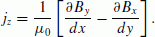(1)

Where μ0 is the magnetic permeability, B x and B y are the horizontal components of the magnetic field after removal of the mean magnetic field from the measurements (hereafter called ‘residual field’).

The mathematical approach used here is the contour integral in the horizontal plane as also described briefly in Ritter and Lühr (2006):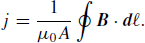(2)

The integral is performed along the closed path of the connecting lines of the quad points. dℓ is a route element along the integration path, B is the residual magnetic field vector (B x , B y , B z ) in the coordinate frame of the flight direction (VHQ, Velocity-Oriented Horizontal Quad, see Section 7), A is the encircled area, and μ0 is the magnetic permeability. The position, where the obtained current density estimate is assigned to, is the centre of the quad. The coding of this approach involves interpolation procedures and simple rotation and subtraction routines. Figure 1 sketches a quad of 4 measurement points, Q1,…, Q4, on the orbit tracks of satellites SwA and SwB, and the integration path encircling the spanned integration area. The current density obtained is a mean value of the current densities within the quad. Also the temporal variations of the currents within the quad points are averaged over about 20 s.

In the paper at hand the integral method used to determine Swarm Level-2 FAC is explained in detail, contrary to Ritter and Lühr (2006) that focused on the description of the curl-B method. The integral method was selected above the curl-B solution, because the requirements concerning the geometry of the four measurement points needed for the calculation of the current density is less stringent. The integration area spanned by the quad points doesn’t necessarily need to be rectangular, while the two components of spatial derivatives in the curl-B approach have to be orthogonal. The integral method also avoids a division by vanishing cross-track horizontal distances near the orbit crossover points. In the curl-B technique with its horizontal gradients this might lead to unrealistically high current density values in this region.

For the determination of FACs the Swarm magnetic field vector data provided by the Level-1b processor, BL1b, are used. They are provided together with positions and times-tamps in the NEC coordinate frame (North-East-Center, see Section 7). The following paragraphs will explain the processing steps further.

### 2.1 Data pre-processing

First, the time series are checked for data gaps, interpolated if gaps are minimal, and/or flagged accordingly. The FACs are computed, as mentioned before, from residual magnetic field vector data. That means, the measured magnetic field is corrected for the core, crustal and magneto-spheric fields at satellite altitude. For calculating the magnetic field at the orbit positions, initially the following models are utilized: IGRF11 (Finlay et al., 2010) for the core field, MF7 (Maus et al., 2007) for the lithospheric field, and part of POMME-6 (Lühr and Maus, 2010) for the external field. The mean field, i.e. the sum of BCOR + BLITBEXT, is subtracted from the measured magnetic field data BL1b: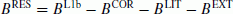(3)

where BCOR is the core magnetic field, BLIT describes the lithospheric magnetization, and BEXT denotes the magnetic field from magnetospheric currents. Subsequently the residual B-field data are low-pass filtered to ensure that only currents with spatial scale lengths > 150 km are represented. This scale length corresponds to the east-west separation of the two satellites at the equator. It is recommended to use a cut-off period of 10–20 sec for this filter to avoid antialiasing. This filter also suppresses Alfvènic wave parts (Ishii et al., 1992) in the data, so that only the stationary part of the FAC is captured by the procedure.

### 2.2 Definition of quad positions and resampling of data

For the estimation of the radial current density a regular quad of measurement points is most suitable. The proper choice of these quads is vital for the quality of the current density. The distance of the quad points in along-track direction should be comparable, at least in the most interesting regions, with the longitudinal separation, dy, of the orbits. This separation is ~150 km at the equator, decreasing at high latitudes and it vanishes near the geographic poles (dy ~ 150 km cos (lat)). Hence the time between along-track quad points was chosen 5 sec corresponding to ~40 km. To avoid collision at the orbit crossovers, the satellites pass the equator with a time separation of 5–10 s. This time separation Δt is taken into account when selecting the readings of symmetric quad points.

The synchronization of SwB with SwA is done for the northern and the southern hemisphere passages separately. The time shift is chosen that the satellites meet virtually at the crossover of their orbits. In this way, quads are defined at sampling intervals of 1 s along the time series of each hemispheric orbit arc. The current positions in the quad centres and time stamps are estimated from the mean of the coordinates (ITRF, Conventional Terrestrial Reference Frame, see Section 7) of all four quad points, e.g.: tfac = (t1 + t2 + t3 + t4)/4.

### 2.3 Transformation of B into directions of route elements

The route elements d i (i = 1 ….4, see Fig. 1(a)) of each quad are determined by the vector differences of the position vectors to each quad point in the local time/latitude (LTL, see Section 7) frame. The LT-related longitude, λ, is estimated using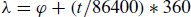(4)

where ϕ is the geocentric longitude.

The route elements are estimated from the Cartesian position vectors: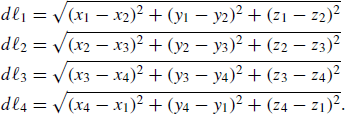(5)

The route elements, dℓ, mark the path from one quad point to the next one; for example, d1 marks the path from point Q1 to point Q2. Figure 3(top) shows the evolution of the route elements’ size on one full orbit. Along-track-route elements along track, d1,3, are constant, whereas those between the satellites, d2,4, are smallest close to the geographic poles, where the satellites’ orbits cross.

In order to determine the magnetic field component in flight direction, the angles between route elements parallel (α) and route elements transverse (β) to flight direction and the respective geographic pole are defined as sketched in Fig. 2 for the satellites’ passage of the northern hemisphere. The equations for deriving the angles α and β take into account the spherical shape of the ionosphere. They are based on spherical geometry and make use of great circle distances. The basic equation is the sine formula for triangles on a sphere: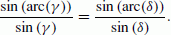(6)

Where arc(γ ) and arc(δ) represent the sides of the triangle opposite to the respective angles γ and δ.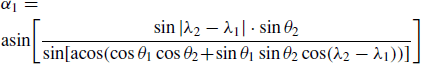(7)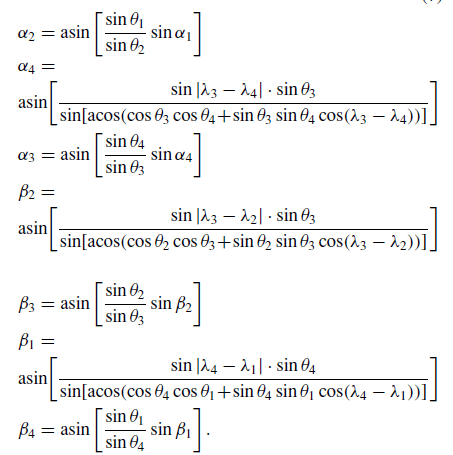(8)

In all equations above, θ i refers to the co-latitude and λ i to the longitude of a given quad point in the LTL frame. In the southern hemisphere, the angles are estimated relative to the South pole. However, the equations above can be used for the processing of either hemisphere, as the sign switches of the angles at the equator. When the satellites cross close to the geographic poles, the angles α and β need to be adapted to account for sign switches of the components. Further details are given in the Detailed Processing Model Document (Swarm Level 2 Processing System Consortium, 2012).

Figure 3(bottom) shows the evolution of angles α and β after this adaption on one full orbit. Both angles vary smoothly and steadily along the orbit. Only β jumps by 180° at the orbit crossings. On the descending arc, α takes values within the range [−90°… 0°… −90°], on the ascending arc, the angles range between [−90°… −180°… −90°]. The angle β ranges from [0°… 90°… 0°] on the descending arc, whereas it takes values of [180°… 90°… 180°] on the ascending arc.

The angles α and β are then used to transform the residual magnetic fields from NEC into the flight direction frame VHQ (Velocity-oriented Horizontal Quad):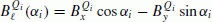(9)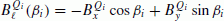(10)

with i = 1,2 for spacecraft SwB and with i = 3, 4 for spacecraft SwA.

B(α i ) is aligned with the axis along flight direction at any one of the quad points Q i . B(β i ) is aligned with the transverse axis of the quad, i.e. the connection line between two orbit-synchronous measurement points of SwA and SwB (see Eqs. (7), (8)). Q i refer to the quad points defined above. The values of B are fed into the integral equation for the determination of the radial current density.

### 2.4 Calculation of radial currents by integration

From the rotated B vector the four product terms P i for the integral can be estimated from the sums of the horizontal fields. We use averages of the magnetic field values at the end points of the route elements: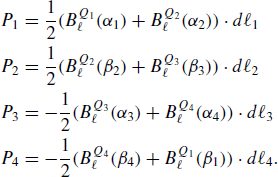(11)

The contour integral (Eq. (2)) is performed along the closed path of the connecting lines of the quad points. dℓ is a route element along the integration path, B is the measured magnetic field vector (B x , B y , B z ) in the coordinate frame of the flight direction (VHQ). Practically the integral is performed by adding the product terms P i :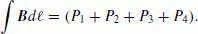(12)

Division by the magnetic permeability, μ0, and the quad area, A, yields the radial current density j r :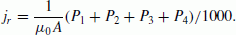(13)

If the route elements, like the position radii, and the enclosed area A are given in [m] and the magnetic field readings in [nT], the values need to be divided by 1000 to obtain the current density in units of [μ A/m2].

The integration area is the area encircled by the route elements dℓ (Fig. 1(a)):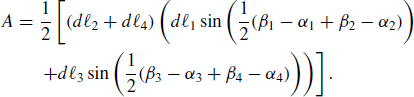(14)

Here, we allow for a certain distortion of the area framed by the symmetric quad. This radial current density is computed at each of the quads at 1 s intervals along the entire orbits. Close to the geographic poles, no radial currents can be estimated if the horizontal spacecraft distance d2 or d4 is too small. Initially, we chose 3 km for the minimum distance of the satellites (Ritter and Lühr, 2006). This is the case for geographic latitudes θ > 86° and causes a data gap of 15 s near the poles, occurring typically in the quiet polar cap region.

### 2.5 Determination of field-aligned currents

The Swarm L1b data is given in the Earth-fixed coordinate system (NEC): i.e. the three coordinate axes are fixed with respect to the rotating Earth’s surface. The radial current, j r , computed in the previous section from these data is also presented in this system. To obtain the current density flowing along the mean magnetic field, i.e. the field-aligned current, this radial current component has to be completed by the inclination of the mean magnetic field. The mean magnetic field vector BMF at each current position is computed as the sum of the core, crustal and magnetospheric model fields in the NEC frame (see Eq. (3)). The mean field inclination is estimated from the above models at each current position: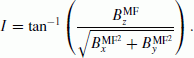(15)

The full field-aligned current density, j, is obtained by dividing the radial current reading, j r , by the sine of the inclination angle: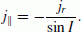(16)

The FAC density comes in units of [μ A/m2]. To avoid unre-alistically large FAC densities around the magnetic equator due to near-horizontal inclinations, no FAC values will be estimated for magnetic field inclinations, ǀIǀ, smaller than 30°, i.e. about ±15° in latitude off the magnetic equator. The radial current densities are reported all the way across the equator and thus can be used to monitor the vertical currents driven by the F-region dynamo.

More details of the processing approach used for estimating the Swarm FAC products can be found in the Detailed Processing Model Document (Swarm Level 2 Processing System Consortium, 2012).

## 3. Determination of the Single-Satellite Solution

The single-satellite FAC processing is done in a separate step. For the simple FAC determination from single-satellite data, the current is generally assumed to flow in current sheets perpendicular to the magnetic meridian. Ideally, the field-aligned current, j is computed from the temporal gradient of the azimuthal magnetic field component and the spacecraft velocity along the meridional component in the mean field-aligned coordinate (MFA, see Section 7) frame: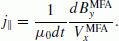(17)

For the actual implementation in the Level-2 processor, we chose a different approach. To avoid singularities of the velocity in the MFA system near the equators, the measurements are transformed into the spacecraft velocity frame (VSC, see Section 7) and the field-aligned currents are assumed to flow in sheets perpendicular to the orbit plane. Obviously the real current geometry may deviate from this ideal setting. However, the simplistic approach described here was chosen above more sophisticated methods such as Minimal Variance Analysis to guarantee provide a fast and reliable automatic data processing. Deviations from the assumption given above are accounted for by the estimation of uncertainties detailed in Section 4. The field gradients and velocities are computed at time spacings of dt = 1 s. In case of the single-satellite solutions, no filtering of the data is performed. Therefore this technique resolves also small-scale structures.

In the VSC frame the two horizontal velocity components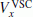and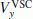are equal: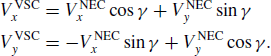(18)

Based on this condition, the rotation angle γ can be derived from the ratio of the difference and sum of the VNEC components:(19)

The angle γ is also used for the transformation of the magnetic field from the NEC into the VSC frame: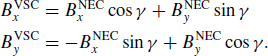(20)

The ionospheric radial current (IRC) density is computed from the horizontal gradients of BVSC (i.e. differences between two successive measurements, e.g.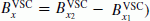and the horizontal velocity components of VVSC (Lühr et al., 1996):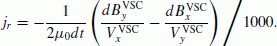(21)

Since the velocities V x and V y have the same values, the magnetic field variations B x and B y are weighted equally (Lühr et al., 1996). If the velocities are given in [m/s] and the magnetic field measurements in [nT], the current estimates are obtain in the units [μ A/m2]. The positions assigned to the currents are the centres between the two measurement points.

As for the multi-satellite solution, the radial current component needs to be completed by the inclination of the magnetic field, as outlined in Eq. (16), to represent the FAC density.

## 4. Uncertainties of Radial and Field-Aligned Currents

The formal uncertainty calculation for the IRC / FACs is based on the following assumptions:

• Biases, bmf, of any magnetic field reading (SwA, SwB, or SwC) are ± 1 nT (Mission Requirements Document, 2004); they do not change during the 5 s interval between two quad point readings;

• Resolutions (digitisation noise), rmf, of any measurement magnetic field reading (SwA, SwB, or SwC) are ±0.1 nT (Mission Requirements Document, 2004);

• Each magnetic field reading has an uncertainty of bmf ± rmf.

• Positions and hence the route elements have no uncertainty (Medium Orbit Determination MOD, ~1 m)

These numbers represent performance characteristics of the Swarm Level-1b data products.

• The two route elements along-track are equally long. So are the two route elements across-track. d1 = d3 and d2 = d4.

For the product terms P i of the integral (Eq. (11)) the uncertainties along the hemispheres are: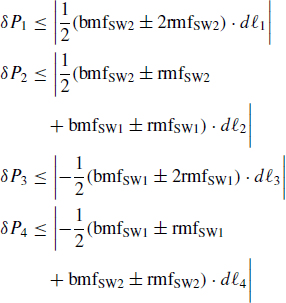(22)

With above assumptions the sum of these terms yields: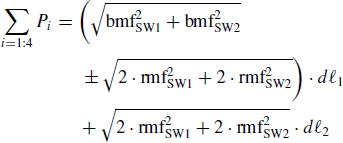(23)

Using the integration area given in Eq. (14) in the simplified form A = d1d2 we obtain for the uncertainty of the integral Eq. (13):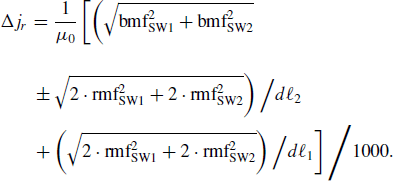(24)

Note that the biases and resolutions of independent measurements are added as squares in the root terms. The second term is constant, since the route element d1 doesn’t vary along the orbit. Hence the variation of the ICR uncertainty depends entirely on the variation of the cross-track route elements d2. The IRC uncertainty is largest in the high latitude regions, where d2 gets very small, and minimal around the equator. The obtained formal uncertainties range from 12 to 430 [nA/m2] (see Fig. 4). Uncertainties of the mean magnetic field models cancel in the integration process, because they are based on scalar potentials.

For the single-satellite solution, an additional uncertainty is due to the unknown orientation of the current sheet. It generally causes an underestimation of the current density. We assume a deficit of 15% on average of the current estimate accounting for a tilt angle up to 45°. With these assumptions the current calculated in Eq. (21) has an uncertainty of: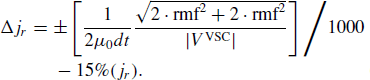(25)

Gradients are estimated from two readings of the same satellite and are assumed to have a resolution of 1 rmf. The velocity components in Eq. (21) are constant along the orbit: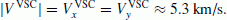(26)

Hence the uncertainty estimate of the single-satellite radial current can be quantified as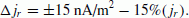(27)

Uncertainties due to the mean magnetic field subtracted in the preprocessing step may also play a role and are estimated to account for 5% of the current density.

The FAC uncertainty is determined by dividing the ICR uncertainty by the sine of the inclination angle: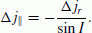(28)

The inclination-induced uncertainty is small in the high latitude regions and increases towards the equator (see Fig. 4).

## 5. Description of the Level-2 IRC/FAC Product

The radial and field-aligned current densities are calculated by the Swarm Level-2 processor as an automatically computed product. The product is provided using the dual-satellite method on the lower pair of satellites SwA and SwB (Swarm L2 product name: FAC_TMS_2F), and the single-satellite solution for each of the Swarm spacecraft SwA, SwB, and SwC individually (Swarm L2 product name: FACxTMS_2F, x=A,B,C). The IRC/FACs data are given with a time resolution of 1 Hz. For the dual-satellite solution the data are filtered, hence the scale size of the resulting current density is >150 km. The unfiltered 1 Hz single-satellite solution has a scale length of >15 km. The dual satellite data product and the three single satellite solutions are provided in separate files. The main part of each of the L2-FAC products consists of timestamp, position, IRC density, FAC density, product flag and Level-1b quality flag. These parameters are listed in Table 1.

The processing Flag (‘Flags’) has 10 individual and independent digits, each of which gives information about a different problem that may have occurred during the processing chain at each position of current density:

Digits 1–8 report problems that may have occurred at one or more of the measurement points used for the computation of a current density value. Values 0-N mark the number of points that were affected by that problem: N = 1… 4 for normal FAC processing (4 measurement points involved),

N = 1… 2 for single-satellite processing (2 measurement points involved).

At each current position, the flag values of the measurement points concerned were added. The values of the digits have the following interpretation:

• 0: default, none of the measurement points involved had the problem and processing was executed normally; the computed current density at that position is good.

• 1…N : One or more measurement points had the problem and a work-around was performed; the computed current density at that position might not be as good as it would be without the problem. It is not important, which one of the points had the problem.

Digits 9–10 report whether the NaN value of the current density at this position is intended or not:

• 0: default; if a NaN occurs at this record, the reason for the NaN is not known and results from computational problems. Normally this should not occur.

• 1: the NaN at this current position is intended: the current position is either near the geographic pole or near the magnetic equator.

Table 2 lists the problems reported by the 10 digits of ‘Flags’.

For each current estimate, the Level-1b flag values of the measurement points concerned were added in the same way, as described for the processing flag.

## 6. Scientific Validation of the Level-2 FAC Product

To validate the radial current algorithm we used the synthetic dataset as employed for the phase A study (Venner-strøm et al., 2005, 2006; Moretto et al., 2006). For generating this test dataset, a global Magneto-Hydro-Dynamics (MHD) model (GGCM, Raeder, 2003) had been run at the Community Coordinated Modeling Centre (CCMC) to simulate the interaction of the solar wind with the magneto-sphere. The resulting field-aligned currents were closed in the ionosphere. By employing an empirical model for the ionospheric conductivity the spatial distribution of the electric potential was deduced, and Hall and Pedersen currents in the ionosphere could be computed. The 3D distribution of magnetic field perturbations generated by these currents was derived. The magnetic field components were then computed at spherical grid points. For a verification of the processing algorithms synthetic magnetic field measurements were derived along predicted Swarm orbits by cubic spline interpolation of the model data on the grid points.

The FAC output of both the dual-satellite method and the single-satellite method (using SWA, SWB and SWC separately) are compared to the FAC densities of the input model of the test dataset. For this purpose the FACs of this input model are sampled along the estimated FAC positions of the data product for validating the current densities resulting from the FAC algorithm.

Figure 5 shows the comparison of the resulting IRC and the model input current at the northern hemisphere. The solid red curve shows the radial current computed by the FAC processor on the 2nd pass across the northern hemisphere on 2000-04-05. The blue curve shows the radial current retrieved from the input model at the computed current positions of the same polar pass for reference. The satellites cross the polar region from the left to the right side. Since the model currents cover only a region down to 60° of latitude, signatures equatorward of 60° are meaningless in terms of currents. The root mean square (rms) of the differences between the computed radial current and the reference current on a profile [±30°] across the geographic North Pole is 28.9 nA/m2. The agreement of the model input and processor result is excellent.

Figure 6 shows that the comparison of results at the southern hemisphere is on a similar high level. The solid red curve shows the radial current computed by the FAC processor on the 3rd pass across the southern hemisphere on 2000-04-05. The root mean square (rms) of the differences between the computed radial current and the reference current on a profile [±30°] across the geographic South Pole is 26.8 nA/m2.

A proper scientific validation of the multi-point FAC determination is not possible because there are no real magnetic field data available that could represent Swarm measurements. This task has to be performed as part of the Swarm validation activity during the early mission phase. During the in-flight operation the multi-satellite FAC estimates will also be compared with the single-satellite FACs (from SWA and/or SWB separately).

In order to give an impression of the size and distribution of field-aligned currents expected along Swarm orbits, we present in Fig. 7 FAC density magnitudes derived from CHAMP magnetic field measurements. Since the range of FAC intensities is so large, a logarithmic scale (log 10) was chosen. Data are from 02 Jan. 2001. The local time of the orbit is 23 h (top panel) and 11 h (bottom panel). As expected, there are strong FACs observed in the auroral regions, larger amplitudes in the southern (summer) hemisphere than in the northern. At middle and low latitudes also FACs are flowing but at much reduced intensity. On the nightside FAC signatures are primarily related to plasma bubbles near the magnetic equator or to mid-latitude ionospheric irregularities (e.g. Medium Scale Travelling. Disturbances (MSTIDs), near 40° gm lat. in Fig. 7). On the dayside at middle latitudes the FAC density is on average somewhat enhanced. Broad peaks are found around 30° of mag. latitude. They coincide reasonably well with the typical positions of the Sq foci where potential differences between the hemispheres are expected to be largest.

This example gives an impression of the rich variety of different FAC sources that can be investigated with the help of the Swarm Level 2 data product described here. These data will be important for correctly characterizing the electrodynamics in the ionosphere.

## 7. Coordinate Frames

In the FAC processing, the following coordinate frames are used:

ITRF: (x, y, z) The IERS Conventional Terrestrial Reference Frame (ITRF) is an Earth-fixed Cartesian system used for describing the orbit ephemeris. The origin of the frame is the Earth’s centre of mass. The x axis points towards the IERS Reference Meridian (close to Greenwich); the z axis points to the Reference North Pole; the y axis completes the triad.

NEC (North-East-Center ITRF): (x, y, z) The x and y components lie in the horizontal plane, pointing northward and eastward, respectively. z points to the centre of gravity of the Earth.

MFA (Mean Field-Aligned): (x, y, z) The MFA frame is a local coordinate system defined by the ambient magnetic field. It is particularly useful for describing electric currents in the topside ionosphere. The origin is the local measurement point of the magnetic field. The z axis is aligned with the unperturbed magnetic field which points from the southern to the northern hemisphere; the y axis is perpendicular to the magnetic meridian pointing predominantly eastward; the x axis completes the triad having an outward component.

VSC (spacecraft velocity): (x, y, z) This frame is introduced for single-satellite FACs calculation. The x and y components lie in the horizontal plane, pointing 45° away from the actual flight direction. z points to the centre of the Earth.

VHQ (Velocity-oriented Horizontal Quad): (α, β) The frame describes the orientation of the route elements with respect to the direction towards the pole in the LTL frame. The angle α describes the angle between an along-track route element and the direction towards the pole, whereas β denotes the angle between a route element transverse to the flight direction and the poleward direction.

LTL (Local Time-Latitude) frame: (r, θ, λ) describe the geocentric position with respect to the local time (LT) frame, where r is the radial distance from the Earth’s centre, θ the colatitudes and λ a local time related longitude.

## References

• Dunlop, M. W., A. Balogh, K.-H. Glassmeier, and P. Robert, Four-point Cluster application of magnetic field analysis tools: The Curlometer, J. Geophys. Res., 107(A11), 1384, doi:10.1029/2001JA005088, 2002.

• Finlay, C. C, S. Maus, C. D. Beggan, T. N. Bondar, A. Chambodut, T. A. Chernova, A. Chuillat, V. P. Golovkov, B. Hamilton, M. Hamoudi, R. Holme, G. Hulot, W. Kuang, B. Langlais, V. Lesur, F. J. Lowes, H. Lühr, S. Macmillan, M. Mandea, S. McLean, C. Manoj, M. Menvielle, I. Michaelis, N. Olsen, J. Rauberg, M. Rother, T. J. Sabaka, A. Tangborn, L. Tøffner-Clausen, E. Thébault, A. W. P. Thomson, I. Wardinksi, Z. Wei, and T. I. Zvereva, International Geomagnetic Reference Field: The eleventh generation, Geophys. J. Int., 183(3), 1216–1230, 10.1111/j.1365-246X.2010.04804.x, 2010.

• Fukushima, N., Electric potential difference between conjugate points in middle latitudes caused by asymmetric dynamo in the ionosphere, J. Geomag. Geoelectr., 31, 401–409, 1979.

• Ishii, M., M. Sugiura, T. Iyemori, and J. A. Slavin, Correlation between magnetic and electric fields in the field-aligned current regions deduced from DE-2 observations, J. Geophys. Res., 97, 13,877, 1992.

• Kan, J. R. and L. C. Lee, Energy coupling function and solar wind-magnetosphere dynamo, Geophys. Res. Lett., 6, 577, 1979.

• Lühr, H. and S. Maus, Solar cycle dependence of quiet-time magneto-spheric currents and a model of their near-Earth magnetic fields, Earth Planets Space, 62(10), 843–848, doi:10.5047/eps.2010.07.012, 2010.

• Lühr, H., J.J. Warnecke, and M. Rother, An algorithm for estimating field-aligned currents from single-spacecraft magnetic field measurements: A diagnostic tool applied to Freja satellite data, IEEE Trans. Geosci. Remote Sens., 34, 1369–1376, 1996.

• Marchaudon, A., J.-C. Cerisier, M. W. Dunlop, F. Pitout, J.-M. Bosqued, and A. N. Fazakerley, Shape, size, velocity and field-aligned currents of dayside plasma injections: A multi-altitude study, Ann. Geophys., 27, 1251–1266, 2009.

• Maus, S. and P. Weidelt, Separating the magnetospheric disturbance magnetic field into external and transient internal contributions using a 1D conductivity model of the Earth, Geophys. Res. Lett., 31(12), L12614, doi:10.1029/2004GL020232, 2004.

• Maus, S., H. Luhr, M. Rother, K. Hemant, G. Balasis, P. Ritter, and C. Stolle, Fifth-generation lithospheric magnetic field model from CHAMP satellite measurements, Geochem. Geophys. Geosyst., 8, Q05013, doi:10.1029/2006GC00152, 2007.

• Mission Requirements Document: Swarm, The Earth’s Magnetic Field and Environment Explorers SW-MD-ESA-SY-001, vs. 1, 2004.

• Moretto, T., S. Vennerstrøm, N. Olsen, L. Rastätter, and J. Raeder, Using global magnetospheric models for simulation and interpretation of Swarm external field measurements, Earth Planets Space, 58, 439–449, 2006.

• Olsen, N., E. Friis-Christensen, R. Floberghagen, P. Alken, C. D Beggan, A. Chulliat, E. Doornbos, J. T. da Encarnação, B. Hamilton, G. Hulot, J. van den IJssel, A. Kuvshinov, V. Lesur, H. Lühr, S. Macmillan, S. Maus, M. Noja, P. E. H. Olsen, J. Park, G. Plank, C. Puthe, J. Rauberg, P. Ritter, M. Rother, T. J. Sabaka, R. Schachtschneider, O. Sirol, C. Stolle, E. Thébault, A. W. P. Thomson, L. Tøffner-Clausen, J. Velímský, P. Vigneron, and P. N. Visser, The Swarm Satellite Constellation Application and Research Facility (SCARF) and Swarm data products, Earth Planets Space, 65, this issue, 1189–1200, 2013.

• Park, J., H. Lühr, and K. W. Min, Climatology of the inter-hemispheric field-aligned current system in the equatorial ionosphere as observed by CHAMP, Ann. Geophys., 29(3), 573–582, doi:10.5194/angeo-29-573-2011, 2011.

• Raeder, J., Global geospace modeling: Tutorial and review, in Space Plasma Simulations, edited by J. Buchner, C. T. Dunn, and M. Scholer, Lecture Notes in Physics, vol. 615, Springer Verlag, Berlin, 2003.

• Ritter, P. and H. Lühr, Curl-B technique applied to Swarm constellation for determining field-aligned currents, Earth Planets Space, 58(4), 463–476, 2006.

• Stauning, P., F Primdahl, J. Watermann, and O. Rasmussen, IMF By related cusp currents observed from the Ørsted satellite and from ground, Geophys. Res. Lett., 28, 99–102, 2001.

• Swarm Level 2 Processing System Consortium, Detailed Processing Model (DPM) FAC, Swarm Level 2 Processing System, SW-DS-GFZ-GS-0002, vs. 2d, 2012.

• Untiedt, J. and W. Baumjohann, Studies of polar current systems using the IMS Scandinavian magnetometer array, Space Sci. Rev., 63, 245–390, 1993.

• Vennerstrøm, S., E. Friis-Christensen, H. Lühr, T. Moretto, N. Olsen, C. Manoj, P. Ritter, L. Rastaetter, A. Kuvshinov, and S. Maus, Swarm— The impact of combined magnetic and electric field analysis and of ocean circulation effects on Swarm Mission performance: Final Report, DSRI Report 2/2004, 2005.

• Vennerstrøm, S., T. Moretto, L. Rastätter, and J. Raeder, Modeling and analysis of solar wind generated contributions to the near-Earth magnetic field, Earth Planets Space, 57, 451–461, 2006.

## Acknowledgments

The authors acknowledge the valuable help and comments by the reviewers. The CHAMP mission was sponsored by the Space Agency of the German Aerospace Center (DLR) through funds of the Federal Ministry of Economics and Technology, following a decision of the German Federal Parliament (grant code 50EE0944). The development of the Swarm L2-FAC prototype was sponsored by the European Space Agency (ESTEC) through contract No. 4000102140/10/NL/JA.

## Author information

Authors

### Corresponding author

Correspondence to Patricia Ritter.

## Rights and permissions

The images or other third party material in this article are included in the article’s Creative Commons licence, unless indicated otherwise in a credit line to the material. If material is not included in the article’s Creative Commons licence and your intended use is not permitted by statutory regulation or exceeds the permitted use, you will need to obtain permission directly from the copyright holder.

To view a copy of this licence, visit https://creativecommons.org/licenses/by/4.0/.

Reprints and Permissions

Ritter, P., Lühr, H. & Rauberg, J. Determining field-aligned currents with the Swarm constellation mission. Earth Planet Sp 65, 1285–1294 (2013). https://doi.org/10.5047/eps.2013.09.006

• Revised:

• Accepted:

• Published:

• Issue Date:

• DOI: https://doi.org/10.5047/eps.2013.09.006

### Key words